SCERT AP 7th Class Maths Solutions Pdf Chapter 7 Ratio and Proportion Ex 7.6 Textbook Exercise Questions and Answers.

## AP State Syllabus 7th Class Maths Solutions 7th Lesson Ratio and Proportion Ex 7.6

Question 1.
A shop selling sewing machines, offers 3% discount on purchases. If the marked price is ₹ 6500. then what is the selling price?
Method 1:
Given the marked price = ₹ 6500
bis count = 3%
Disount calculated on marked price.
Discount = 3% of ₹ 6500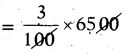∴ Discount = ₹ 195

Selling price
= Marked price – Discount = 6500 – 195
∴ Selling price of sewing machine
= ₹ 6305

Method 2 :
M.P = 100% = ₹ 6500
S.P at a discount of 3%
= (100 – 3)% =?
So, if 100% = 6500
97% = ?
S.P = $$\frac{97}{100}$$ × 6500 = ₹ 6305Question 2.
The marked price of a ceiling fan is ₹ 720 during off season, it is sold for ₹ 684. Determine the discount percentage.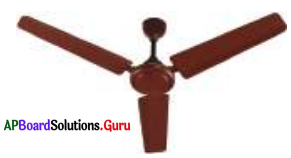Given marked price = ₹ 720
Selling price = ₹ 684
Discount = M.P – S.P = 720 – 684
= ₹ 36
Discount percent = $$\frac{\text { Discount }}{\mathrm{MP}}$$ × 100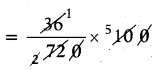= 5%
∴ Discount percent = 5%

Question 3.
A publisher gives 32% discount on the printed price of books to book sellers. If the printed price is ₹ 275, then what amount does the seller has to pay to publisher?
Sol. Given printed (marked) price = ₹ 275
Discount percent = 32%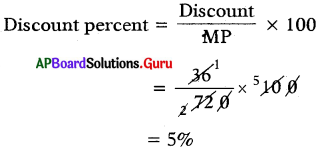Selling price = M.P – Discount = 275 – 88
∴ Selling price of books = ₹ 187Question 4.
Rohit buys an item at 25% discount on the marked price. If he bought it for ₹ 660, what is the marked price?
Method 1 :
Given selling price = ₹ 660
Discount percentage = 25%
Let the marked price = ₹ x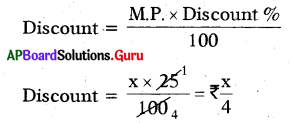Marked price – Selling price = Discount
x – 660 = $$\frac{x}{4}$$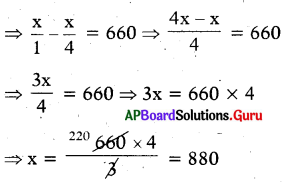∴ Marked price = ₹ 880

Method 2 : M.P = 100% (say)
S.P at a discount of 25% means
S.P = (100 – 25) = 75%
∴ If 75% = ₹ 660 then 100% =?
⇒ SP = $$\frac{100}{75}$$ × 660 = ₹ 880